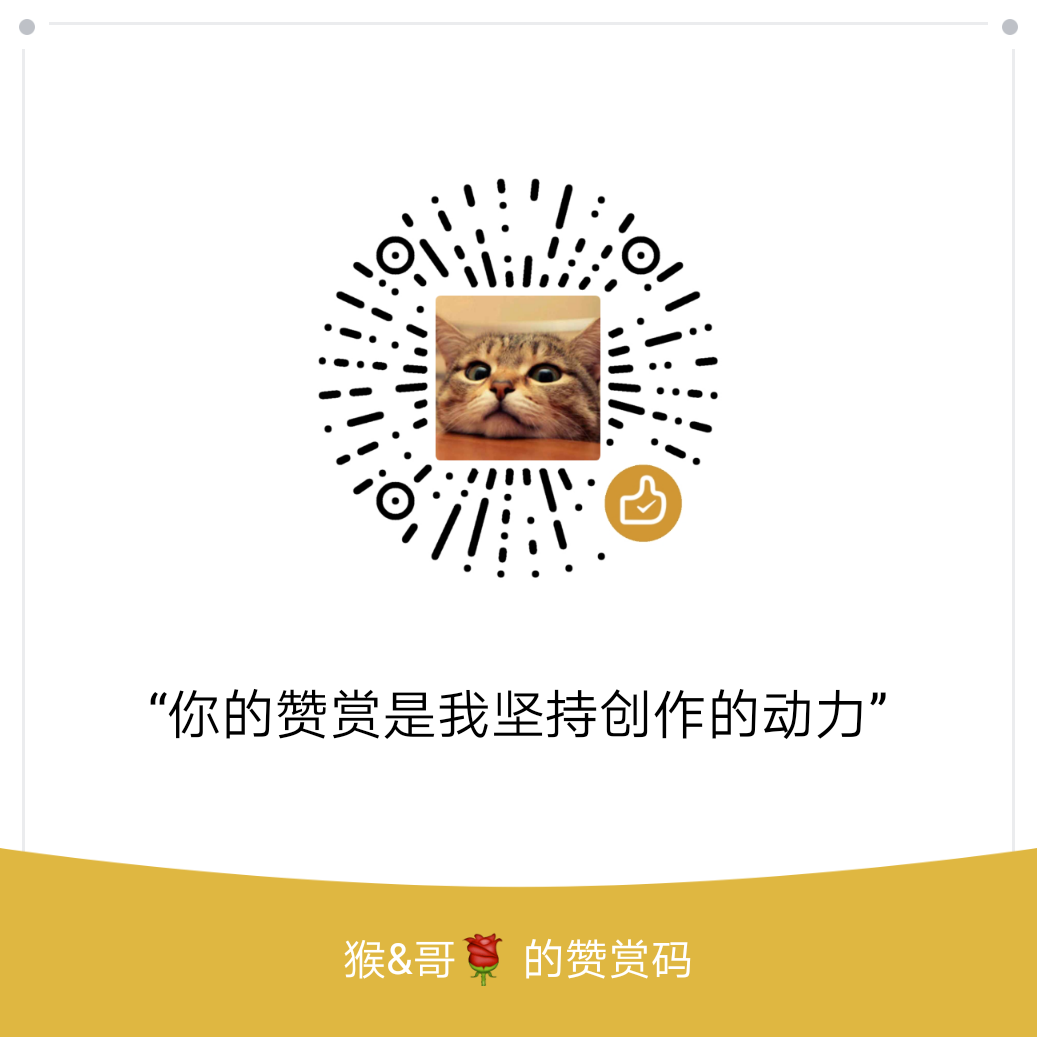• 欢迎访问极客猴，分享 Python 入门，网络爬虫，数据分析，赚钱思维相关的技术与思维`QQ群`
• 本站点文章首发于微信公众号【极客猴】，欢迎关注，第一时间接受文章推送~
• 如果您觉得本站非常有看点，那么赶紧使用 Ctrl+D 收藏极客猴吧

# Django 学习笔记之模型高级用法(下)

3年前 (2018-04-27) 208次浏览

## 1.模型的元数据Meta

``````class Author(models.Model):
name = models.CharField(max_length=40)
email = models.EmailField()

class Meta:
managed = True
db_table = 'author'``````

abstract: 如果 abstract = True，模型会指定为抽象模型。它相当于面向对象编程中的抽象基类。

proxy：如果设置了proxy = True，表示使用代理模式的模型继承方式。

db_table：指定当前模型在数据库的表名。

managed：该属性默认值为 True，表示能创建模型和操作数据库表。

ordering：指定该模型生成的所有对象的排序方式。默认按升序排列，如果在字段名前加上字符 “-” 则表示按降序排列，如果使用字符问号 “？” 表示随机排列。

``````ordering = ['pub_date']             # 表示按'pub_date'字段进行升序排列
ordering = ['-pub_date']            # 表示按'pub_date'字段进行降序排列
ordering = ['-pub_date', 'author']  # 表示先按'pub_date'字段进行降序排列，再按`author`字段进行升序排列。``````

verbose_name：给模型设置别名。如果不指定它，Django 会使用小写的模型名作为默认值。

``````verbose_name = "book"
verbose_name = "图书"``````

verbose_name_plural：因为英语单词有单数和复数两种形式，这个属性是模型对象的复数名。中文则跟 verbose_name 值一致。如果不指定该选项，那么默认的复数名字是 verbose_name 加上 ‘s’ 。

``````verbose_name_plural = "books"
verbose_name_plural = "图书"``````

indexes：为当前模型建立索引列表。用法如下：

``````from django.db import models

class Customer(models.Model):
first_name = models.CharField(max_length=100)
last_name = models.CharField(max_length=100)

class Meta:
indexes = [
models.Index(fields=['last_name', 'first_name']),
models.Index(fields=['first_name'], name='first_name_idx'),
]``````

## 2.模型的继承

### 2-1.抽象模型

``````from django.db import models

# 抽象模型
class Person(models.Model):
name = models.CharField(max_length=500)
age = models.PositiveIntegerField()

class Meta:
abstract = True

# 子模型
class Student(Person):
school_name = models.CharField(max_length=20)``````

### 2-2.多表继承

``````from django.db import models

# 父模型 one
class Model_One(models.Model):
attr1 = models.CharField(max_length=10)

# 父模型 two
class Model_Two(models.Model):
attr2 = models.CharField(max_length=10)

# 子模型
class Multiple(Model_One, Model_Two):
attr3 = models.CharField(max_length=10)``````

### 2-3.代理模型

``````from django.db import models
from django.contrib.auth.models import User

class Person(User):
name = models.CharField(max_length=10)

class Meta:
proxy = True

def do_something(self):
pass

class Man(Person):
job = models.CharField(max_length=20)

class Woman(Person):
makeup = models.CharField(max_length=20)``````[赞赏码]